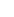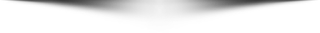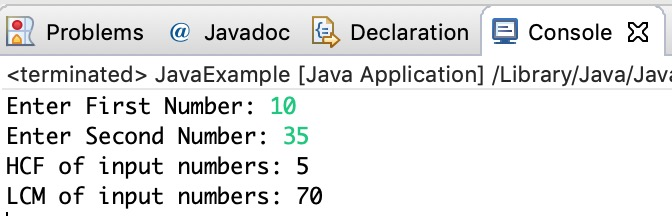# ▹ HOME▹ MUSIC▹ VIDEOS▹ NEWS▹ GOSSIP▹ LIFE STYLE▹ BLOGGING TIPS▹ 18+▹ GUEST POSTARUWAAB9JA HOT ARTISTSSubscribe to our YouTube channel - HereOwn A Blog Like "Aruwa_Ab 9ja"
Page | Group - Follow us - Call us - Hire Us - [email protected]# Java Program to Find HCF and LCM of Two Numbers

Prompting you the best.....

In this tutorial, we will write a java program to find the HCF and LCM of two entered numbers.

LCM (Least Common Multiple): The LCM of two numbers is the smallest positive integer which is divisible by both numbers.
HCF (Highest common Factor): HCF is also known as Greatest common divisor, HCF of two numbers is the largest positive integer that divides both the numbers.

## Program to Find HCF and LCM of Two Numbers in Java

```import java.util.Scanner;

public class JavaExample{
public static void main(String args[]){
int temp1, temp2, num1, num2, temp, hcf, lcm;
Scanner scanner = new Scanner(System.in);

System.out.print("Enter First Number: ");
num1 = scanner.nextInt();
System.out.print("Enter Second Number: ");
num2 = scanner.nextInt();
scanner.close();

temp1 = num1;
temp2 = num2;

while(temp2 != 0){
temp = temp2;
temp2 = temp1%temp2;
temp1 = temp;
}

hcf = temp1;
lcm = (num1*num2)/hcf;

System.out.println("HCF of input numbers: "+hcf);
System.out.println("LCM of input numbers: "+lcm);
}
}```

Output: